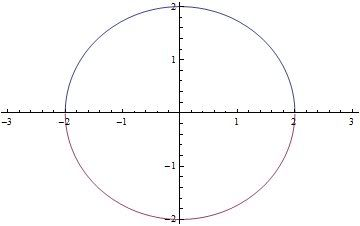# Thread: volume of paraboloid

1. ## volume of paraboloid

find the volume of the paraboloid z=x^2+y^2 below the plane z=4.

i am stuck on setting up the integral..can any one help???

2.Originally Posted by chris25find the volume of the paraboloid z=x^2+y^2 below the plane z=4.

i am stuck on setting up the integral..can any one help???
Let the cross section of the paraboloid at z=4 be our region R.

Thus, the x and y limits of integration become pretty obvious: $\displaystyle \left\{R: \ -2\leq x \leq 2, \ -2\leq y \leq 2\right\}$.(this is the region R)

Thus, our volume integral would be $\displaystyle \int_{-2}^2\int_{-2}^2 x^2+y^2 \,dx\,dy$.

We can also represent this integral in polar coordinates. Note that the limits of integration change!

This time we see : $\displaystyle \left\{R: \ 0\leq r \leq 2, \ 0\leq \theta \leq 2\pi\right\}$.

As a result, the integral becomes $\displaystyle \int_0^{2\pi}\int_0^2 r^3 \,dr\,d\theta$.

Hope that this makes sense!

--Chris

3. Hey, Chris answered Chris!Originally Posted by Chris L T521Let the cross section of the paraboloid at z=4 be our region R.

Thus, the x and y limits of integration become pretty obvious: $\displaystyle \left\{R: \ -2\leq x \leq 2, \ -2\leq y \leq 2\right\}$.(this is the region R)

Thus, our volume integral would be $\displaystyle \int_{-2}^2\int_{-2}^2 x^2+y^2 \,dx\,dy$.

We can also represent this integral in polar coordinates. Note that the limits of integration change!

This time we see : $\displaystyle \left\{R: \ 0\leq r \leq 2, \ 0\leq \theta \leq 2\pi\right\}$.

As a result, the integral becomes $\displaystyle \int_0^{2\pi}\int_0^2 r^3 \,dr\,d\theta$.

Hope that this makes sense!

--Chris
actually, wouldn't the volume be given by $\displaystyle V = \int _{-2}^2 \int_{- \sqrt{4 - x^2}}^{\sqrt{4 - x^2}} \int_{x^2 + y^2}^4 ~dzdydx= \int _{-2}^2 \int_{- \sqrt{4 - x^2}}^{\sqrt{4 - x^2}} \bigg[4 - (x^2 + y^2) \bigg]~dydx$

which yields $\displaystyle \int_0^{2 \pi} \int_0^2 (4r - r^3)~dr d \theta$ ?

...or am iagain?

#### Search Tags

paraboloid, volume